# Quantity and Rate Analysis for Reinforced Concrete ConstructionReading time: 1 minute

Today we will see how to prepare rate analysis for Reinforced Concrete (RCC) work. First step to rate analysis is the estimation of labour, materials, equipments and miscellaneous items for particular quantity of reinforced concrete. The second step is to determine the component of structure for which the RCC rate analysis is required, as the quantity of reinforcement steel varies with slabs, beams, columns, foundation, RCC Roads etc., though the quantity of other materials like sand, coarse aggregate and cement remain the same with the same mix design (mix proportion) of concrete. Labour rates for reinforcement work changes with type of structural component as the quantity of reinforcement steel changes. The Quantity of materials like sand, cement and coarse aggregates vary with mix design such as M15 (1:2:4), M20 (1:1.5:3), M25, M30 etc..Here we will see the rate analysis for 1m3 of reinforced concrete.

## Data required for RCC Rate Analysis:

### 1. Estimation of materials:

Material estimation include sand, cement, coarse aggregate and steel for a particular mix design. Let us consider a mix design of 1:1.5:3 for our estimation practice. The dry volume of total materials required is considered as 1.54 times the wet volume of concrete, due to voids present in sand and aggregates in dry stage. Therefore, for our calculation, we will consider the total volume of materials required as 1.54 m3 for 1 m3 of wet concrete.

### a) Bags of cement required:

Volume of cement required for 1m3 of Concrete =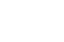=0.28 m3 Then number of bags of cement (volume of one bag of cement = 0.0347 m3) =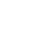= 8.07 bags of cement. b) Volume of Sand required: Volume of sand required =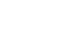= 0.42 m3 of sand.

### c) Volume of Coarse Aggregate Required

Volume of Coarse Aggregate =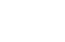= 0.84 m3 of coarse aggregates.

### d) Estimation of Reinforced Steel:

Quantity of steel required depends on components of structure, i.e. slabs, beams, columns, foundations, roads etc. To estimate the steel required, there are two methods. First method is, when we have the drawing available, we can calculate the total weight of steel required divided by total volume of concrete for different components. This will give us the weight of reinforcement steel per cubic meter of concrete. Second method is assuming the percentage of reinforcement for different components. Following are the percentage of reinforcement steel generally required per different components. Its values can vary from structure to structure, and can be assumed from past experiences of similar structure.
• For slabs = 1.0 % of concrete volume.
• For Beam = 2 % concrete volume.
• For column = 2.5 % of concrete volume.
• For RCC Roads, 0.6% concrete volume.
Lets take example of RCC Column, where reinforcement required is 2.5% of concrete volume, weight of steel required will be: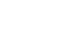=196.25 kg.

### 2. Labour Requirement for 1m3 of RCC:

Labours required are presented in terms of days required by particular labour to complete its work for the given quantity of concrete. Following are the various labours required: a) Mason: As per Standard Schedule of Rates and Analysis of Rates, One mason is required for 0.37 days. b) Labours: One Unskilled labours required for 3.5 days. c) Water carrier: One water carrier required for 1.39 days. d) Bar Bender: Bar bender requirement depends on weight of reinforcement. Lets consider one bar bender required for 100 kg of steel as for 1 day. e) Mixer Operator: One mixer operator required for 0.0714 days. f) Vibrator Operator: One vibrator operator required for 0.0714 days.

### 3. Equipments and sundries:

Equipment and other charges, such as water charges, miscellaneous items, tools and tackles etc can be assumed as some percentage of total cost of materials and labours. Lets say it as 7.5%.

### 4. Contractor’s Profit:

Contractor’s profit depends on place to place, organization to organization and work to work. It varies from 10 – 20%. For our case lets assume it as 15% of total cost of materials, labours and equipments. We have calculated the quantity of every item in above 1 – 3 steps. For rate analysis of RCC, we need to multiply each quantity with their rates to get the amount for every item of work. Rates vary from place to place and time to time. It is advisable to assume local rates or standard rates of the place. The sum total of all the four items above will give the rate or cost for 1m3 of concrete. DOWNLOAD: RCC Rate Analysis Spreadsheet Read More: Rate Analysis of Civil Works -Elements and Requirements Rate Analysis of Plastering with Cement Mortar -Quantity Calculation Rate Analysis for Cement Mortar Quantity of Cement & Sand Calculation in Mortar Analysis of Rates for Civil Engineering Works Rate Analysis for Brick Masonry